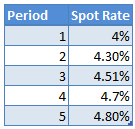# Arbitrage-free Valuation Approach for Bonds

The simplest way to calculate the value of a bond is to take the cash flows of the bond till its maturity and then discount them by a single discount rate. The method is quick but not very accurate because the yield curve is not flat and the interest rates are different for different maturities. A better way to price the bonds is to discount each cash flow with the spot rate (zero coupon rate) for its respective maturity. This is called the arbitrage-free valuation approach. According to this approach the value of a Treasury bond based on spot rates must be equal to the sum of the present values of all cash flows. If the values are not equal, then there is an arbitrage opportunity that can be exploited by an arbitrageur.

Example 1

Let’s take an example. Suppose we want to calculate the value of a $1000 par, 5% coupon, 5-year maturity bond. We also have the following spot rates for the next 5 years:Assuming this is an annual pay bond, the bond will have the following cash flows. Year 1:$50

Year 2: $50 Year 3:$50

Year 4: $50 Year 5:$1000 + $50 The value of the bond can be calculated by discounting these cash flows by their respective spot rate. Bond Value = 50/(1.04)^1+50/(1.0430)^2+50/(1.0451)^3+50/(1.047)^4+1050/(1.048)^5 Bond Value =$1010.033

If the market price of this bond is $1,020, then there is an arbitrage opportunity. The arbitrageur can buy the individual cash flows (in the form of zero-coupon bonds), combine then into a 5-year bond, and sell the package at the market price of the bond. The arbitrageur will earn a risk-free profit of$9.967 ($1020 -$1010.033)

Example 2

Let’s take another example. Suppose we have a bond that matures in 2 years, that has a coupon rate of 6%, and pays coupon semi-annually. The spot rates are 3.9% for 6 months, 4% for 1 year, 4.15% for 1.5 years, and 4.3% for 2 years.

The cash flows from this bond are $30,$30, $30, and$1030.

The value of the bond will be calculated as follows:

Bond value = $30/(1+3.9%/2)^1+$30/(1+4%/2)^2+$30/(1+4.15%/2)^3+$1030/(1+4.3%/2)^4

Bond value = \$1032.45

You can use the above formula to value any bond with any maturity. All you need is the spot rate for the respective maturity.

We can compare this bond value with the market price of the bond to identify arbitrage opportunity.